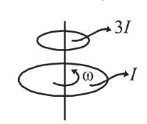# A wheel is rotating freely with an angular speed omega on a shaft.Question:

A wheel is rotating freely with an angular speed $\omega$ on a shaft. The moment of inertia of the wheel is I and the moment of inertia of the shaft is negligible. Another wheel of moment of inertia 3I initially at rest is suddenly coupled to the same shaft. The resultant fractional loss in the kinetic energy of the system is :

1. $\frac{5}{6}$

2. $\frac{1}{4}$

3. 0

4. $\frac{3}{4}$

Correct Option: 4,

Solution:

(4) By angular momentum conservation, $L_{c}=L_{f}$

$\omega I+3 I \times 0=4 I \omega^{\prime} \Rightarrow \omega^{\prime}=\frac{\omega}{4}$$(K E)_{i}=\frac{1}{2} I \omega^{2}$

$(K E)_{f}=\frac{1}{2}(3 I+I) \omega^{\prime 2}$

$=\frac{1}{2} \times(4 I) \times\left(\frac{\omega}{4}\right)^{2}=\frac{I \omega^{2}}{8}$

$\Delta K E=\frac{1}{2} I \omega^{2}-\frac{1}{8} I \omega^{2}=\frac{3}{8} I \omega^{2}$

$\therefore$ Fractional loss in K.E. $=\frac{\Delta K E}{K E_{l_{i}}}=\frac{\frac{3}{8} I \omega^{2}}{\frac{1}{2} I \omega^{2}}=\frac{3}{4}$.